## Tuesday, April 23, 2019

### Generalized Autoregressive Score (GAS) Models: EViews Plays with Python

Starting with EViews 11, users can take advantage of communication between EViews and Python. This means that workflow can begin in EViews, switch over to Python, and be brought back into EViews seamlessly. To demonstrate this feature, we will use U.S. macroeconomic data on the unemployment rate to fit a GARCH model in EViews, transfer the data over and estimate a GAS model equivalent of the GARCH model in Python, transfer the data back to EViews, and compare the results.

### GAS Models

Historically, time varying parameters have received an enormous amount of attention and the literature is saturated with numerous specifications and estimation techniques. Nevertheless, many of these specifications are often difficult to estimate, such as the family of stochastic volatility models, among which GARCH is a canonical example. In this regard, Creal, Koopman, and Lucas (2013) and Harvey (2013) proposed a novel family of time-varying parametric models estimated using the familiar maximum likelihood framework with the score of the conditional density function driving the updating mechanism. The family has now come to be known as the generalized autoregressive score (GAS) family or model.

GAS models are agnostic as to the type of data under consideration as long as the score function and the Hessian are well defined. In particular, the model assumes an input vector of random variables at time $t$, say $\pmb{y}_{t} \in \mathbf{R}^{q}$, where $q=1$ if the setting is univariate. Furthermore, the model assumes a conditional distribution at time $t$ specified as: $$\pmb{y}_{t} | \pmb{y}_{1}, \ldots, \pmb{y}_{t-1} \sim p(\pmb{y}_{t}; \pmb{\theta}_{t})$$ where $\pmb{\theta}_{t} \equiv \pmb{\theta}_{t} (\pmb{y}_{1}, \ldots, \pmb{y}_{t-1}, \pmb{\xi}) \in \Theta \subset \mathbf{R}^{r}$ is a vector of time varying parameters which fully characterize $p(\cdot)$ and are functions of past data and possibly time invariant parameters $\pmb{\xi}$.

What distinguishes GAS models from the rest of the literature is that dynamics in $\pmb{\theta}_{t}$ are driven by an autoregressive mechanism augmented with the score of the conditional distribution of $p(\cdot)$. In particular, $$\pmb{\theta}_{t+1} = \pmb{\omega} + \pmb{A}\pmb{s}_{t} + \pmb{B}\pmb{\theta}_{t}$$ where $\pmb{\omega}, \pmb{A},$ and $\pmb{B}$ are matrix coefficients collected in $\pmb{\xi}$, and $\pmb{s}_{t}$ is a vector proportional to the score of $p(\cdot)$: $$\pmb{s}_{t} = \pmb{S}_{t}(\pmb{\theta}_{t}) \pmb{\nabla}_{t}(\pmb{y}_{t}, \pmb{\theta}_{t})$$ Above, $\pmb{S}_{t}$ is an $r\times r$ positive definite scaling matrix known at time $t$, and $$\pmb{\nabla}_{t}(\pmb{y}_{t}, \pmb{\theta}_{t}) \equiv \frac{\partial \log p(\pmb{y}_{t}; \pmb{\theta}_{t})}{\partial \pmb{\theta}_{t}}$$ It turns out that different choices of $\pmb{S}_{t}$ produce different GAS models. For instance, setting $\pmb{S}_{t}$ to some power $\gamma > 0$ of the information matrix of $\pmb{\theta}_{t}$ will change how the variance of $\pmb{\nabla}_{t}$ impacts the model. In particular, consider: $$\pmb{S}_{t} = \pmb{\mathcal{I}}_{t}(\pmb{\theta}_{t})^{-\gamma}$$ where $$\pmb{\mathcal{I}}_{t}(\pmb{\theta}_{t}) = E_{t-1}\left\{ \pmb{\nabla}_{t}(\pmb{y}_{t}, \pmb{\theta}_{t}) \pmb{\nabla}_{t}(\pmb{y}_{t}, \pmb{\theta}_{t})^{\top} \right\}$$ Typical choices for $\gamma$ are 0, 1/2, and 1. For instance, if $\gamma=0$, $\pmb{S}_{t} = \pmb{I}$ and no scaling occurs. Alternatively, when $\gamma = 1/2$, the scaling results in $Var_{t-1}(\pmb{s}_{t}) = \pmb{I}$; in other words, standardization occurs.

Regardless of the choice of $\gamma$, $\pmb{s}_{t}$ is a martingale difference with respect to the distribution $p(\cdot)$, and $E_{t-1}\left\{ \pmb{s}_{t} \right\} = 0$ for all $t$. This latter property further implies that $\pmb{\theta}_{t}$ is in fact a stationary process with long-term mean value $(\pmb{I}_{t} - \pmb{B})^{-1}\pmb{\omega}$, whenever the spectral radius of $\pmb{B}$ is less than one. Thus, $\pmb{\omega}$ and $\pmb{B}$ are respectively responsible for controlling the level and the persistence of $\pmb{\theta}_{t}$, whereas $\pmb{A}$ controls for the impact of $\pmb{s}_{t}$. In other words, $\pmb{s}_{t}$ denotes the direction of updating $\pmb{\theta}_{t}$ to $\pmb{\theta}_{t+1}$, acting as the the steepest ascent algorithm for improving the model's local fit.

With the above frameowrk established, Creal, Koopman, and Lucas (2013) show that various choices for $p(\cdot)$ and $\pmb{S}_{t}$ lead to various GAS specifications, some of which reduce to very familiar and well established existing models. For instance, let $y_{t} = \sigma_{t}\epsilon_{t}$, and suppose $\epsilon_{t}$ is a Gaussian random variable with mean zero and unit variance. It is readily shown that setting $S_{t} = \mathcal{I}_{t}^{-1}$ and $\theta_{t} = \sigma_{t}^{2}$, the GAS updating equation reduces to: $$\theta_{t+1} = \omega + A(y_{t}^{2} - \theta_{t}) + B\theta_{t}$$ which is equivalent to the standard GARCH(1,1) model $$\sigma_{t+1}^{2} = \alpha + \beta y_{t}^{2} + \eta \sigma_{t}^{2}$$ where $\alpha = \omega$, $\beta = A$, and $\eta = B - A$. There is of course a number of other examples and configurations, and we refer the reader to the original texts for more details.

### Example Description

Our objective here is to communicate between EViews and Python to estimate a GAS model in Python and compare the results back in EViews. In particular, we will work with U.S. monthly civil unemployment rate, defined as the number of unemployed as a percentage of the labor force -- Labor force data are restricted to people 16 years of age and older, who currently reside in 1 of the 50 states or the District of Columbia, who do not reside in institutions (e.g., penal and mental facilities, homes for the aged), and who are not on active duty in the Armed Forces. See the FRED database at https://fred.stlouisfed.org/series/UNRATE) -- to which we will fit a GARCH(1,1) model using the traditional method as well as the GAS approach.

It is well known that unemployment rates are typically very volatile and persistent, particularly in contractionary economic cycles. This is because major firm decisions, such as workforce expansions and contractions, are often accompanied by large sunk costs (e.g. job advertisements, screening, training), and are usually irreversible in the immediate short term (e.g. wage frictions such as labour contracts and dismissal costs). Thus, in contractionary periods, firms typically prefer to defer hiring decisions until more favourable conditions return, resulting in strong unemployment persistence known as spells. On the other hands, these periods are often characterized by frequent labour force transitions and increased search activities, both of which contribute to unemployment volatility.

In light of the above, measuring the volatility of unemployment requires the use of econometric models which are designed to capture both volatility and persistence. While several such models exist in the literature, here we focus on perhaps the most well known such model proposed by Engle (1982) and Bollerslev (1986), the generalized autoregressive conditional heteroskedasticity (GARCH) model described earlier. In particular, if we let $y_{t}$ denote the monthly unemployment rate, we are interested in obtaining an estimate $\widehat{\sigma}_{t}$ of $\sigma_{t}$, at each point in time, effectively tracing the evolution of unemployment volatility for the period under consideration. Since the GAS model above reduces to the GARCH model when the conditional distribution $p(\cdot)$ is Gaussian and the time varying parameter is the volatility of the process, we would like to compare the estimates from the GAS model to those generated by EViews' internal GARCH estimation. Note here that while EViews can estimate numerous (G)ARCH models, it cannot yet natively estimate GAS models. Accordingly, we will fit a GARCH model in EViews, transfer our data over to Python, and estimate a GAS model using the Python package PyFlux. We will then compare our findings.

### Preparatory Work

Before getting started, please make sure that you have Python 3 installed from https://www.python.org/downloads/release/python-368/ on your system, and that you also have the following Python packages installed:
1. NumPy
2. Pandas
3. Matplotlib
4. Seaborn
5. PyFlux
One (certainly not the only) way to install said packages, is to open up a command prompt on your system and navigate to the directory where Python was installed; this is usually C:\Users\USER_NAME\AppData\Local\Programs\Python\Python36_64 if you have a 64-bit version. From there, issue the following commands:
    python -m pip install --upgrade pip
python -m pip install PACKAGE_NAME

Next, make sure that the path to Python is specified in your EViews options. Specifically, in EViews, go to Options/General Options... and on the left tree select External program interface and ensure that Home Path is correctly pointing to the directory where Python is installed. Usually, you will not have to touch this setting since EViews populates this field by searching your system for the install directory.

Finally, please note that as of writing, the analysis that follows was tested with Python version 3.6.8 and PyFlux version 0.4.15.

### Data Analysis in EViews

Turning to data analysis, in EViews, create a new monthly workfile. To do so, click on File/New/Workfile. Under Frequency select Monthly, and set the Start date to 2006M12 and the End date to 2013M12, and hit OK. Next, fetch the unemployment rate data from the FRED database by clicking on File/Open/Database.... From here, select FRED Database from the Database/File Type dropdown, and hit OK. This opens the FRED database window. To get the series of interest from here, click on the Browse button. This opens a new window with a folder-like overview. Here, click on All Series Search and then type UNRATE in the Search For textbox. This will list a series called Civilian Unemployment Rate (M,SA,%). Drag the series over to the workfile to make it available for analysis. This will fetch the series UNRATE from the FRED database and place it in the workfile. In particular, we are grabbing data from the period of December 2006 to December 2013 -- effectively the recessionary period characterized by the recent housing loan crisis in the United States.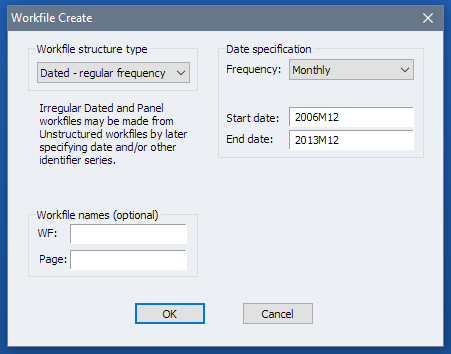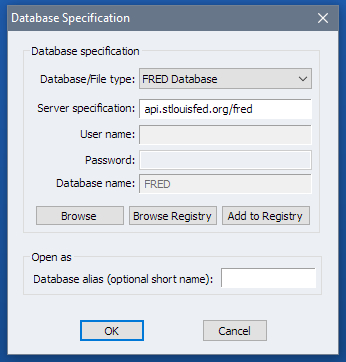Figure 1A: Workfile Dialog Figure 1B: Database Dialog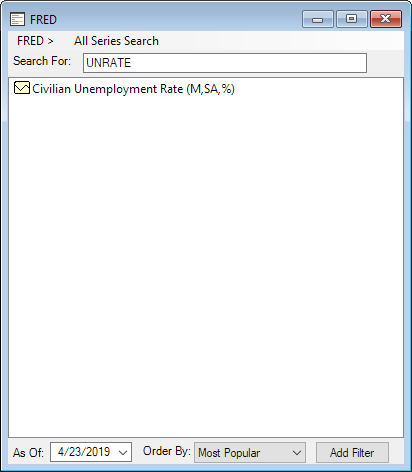Figure 1C: FRED Browse Figure 1C: FRED Search
Also, restrict the sample to the period from January 2007 to December 2013. Why we do this will become apparent later. To do so, issue the following command in EViews:
    smpl 2007M01 @last

To see what the data looks like, double click on a UNRATE in the workfile to open the series object. Next, click on View/Graph.... This will open a graph options window. We will stick with the defaults so click on OK. The output is reproduced below.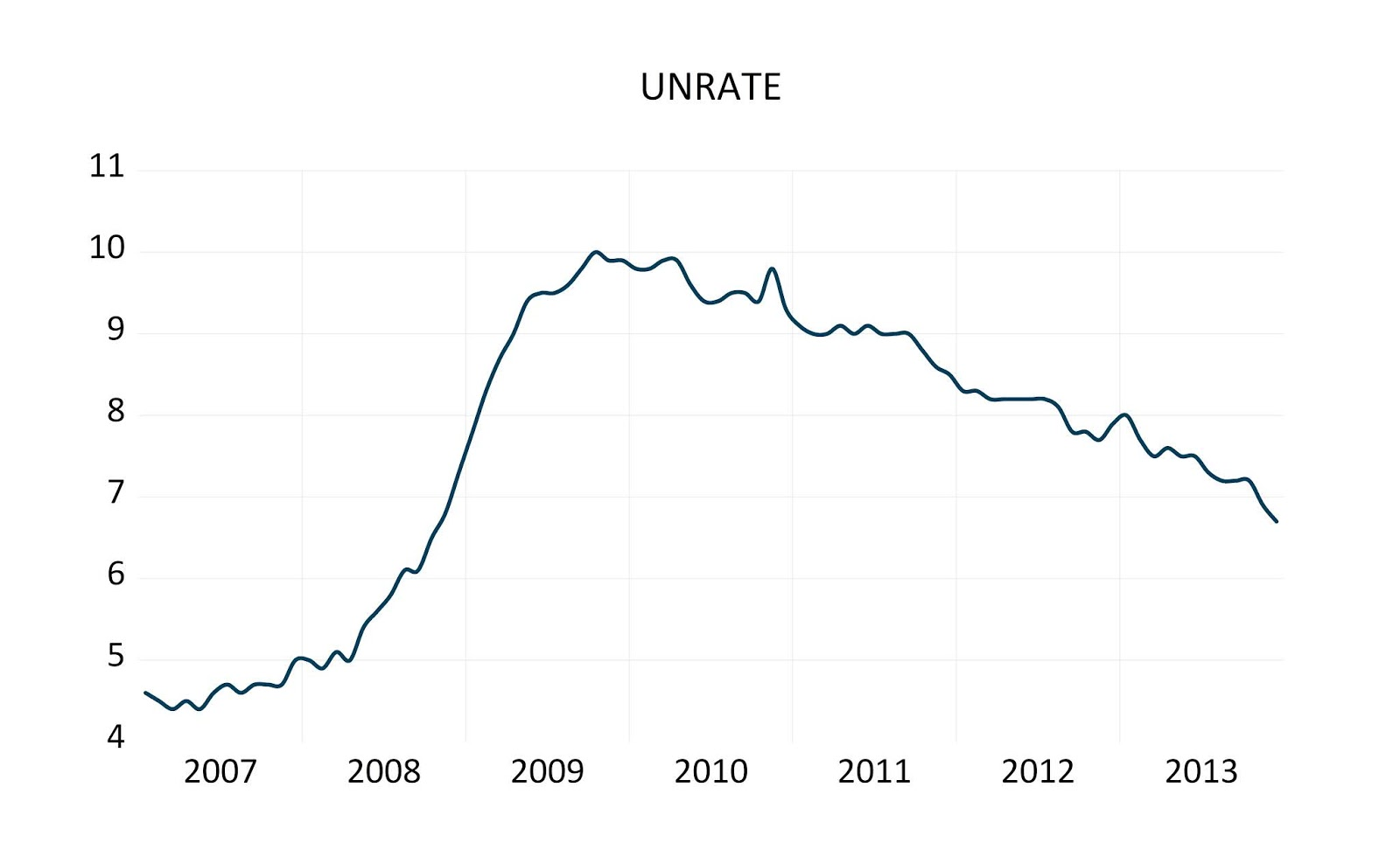Figure 2: Time Series Plot of UNRATE

We will now estimate a basic GARCH model on UNRATE. To do this, click on Quick/Estimate Equation..., and under Method choose ARCH - Autoregressive Conditional Heteroskedasticity. In the Mean Equation text box type UNRATE and leave everything else as their default values. Click on OK.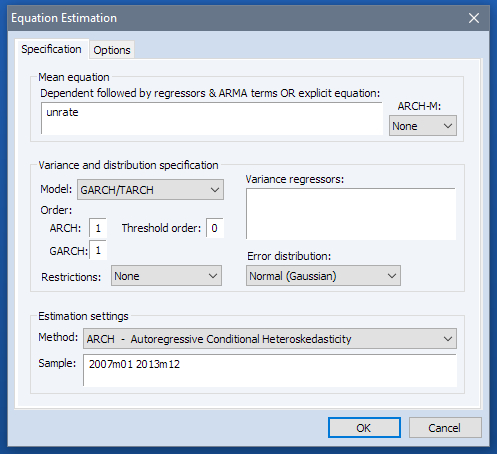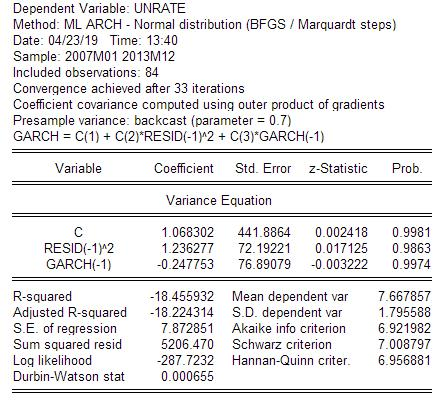Figure 3A: GARCH Estimation Dialog Figure 3B: GARCH Estimation Output
From the estimation output we can see that model parameters have the following estimates:
1. $\alpha = 1.068302$
2. $\beta = 1.236277$
3. $\eta = -0.247753$
We can also see the path of the volatility process by clicking on View/Garch Graph/Conditional Variance. This produces a plot of $\widehat{\sigma}^{2}_{t}$. In fact, we will also create a series object from the data points used to produce the GARCH conditional variance. To do this, from the GARCH conditional variance window, click on Proc/Make GARCH Variance Series... and in the Conditional Variance textbox enter EVGARCH and hit OK. This produces a series object called EVGARCH and places it in the workfile. We will use it a bit later.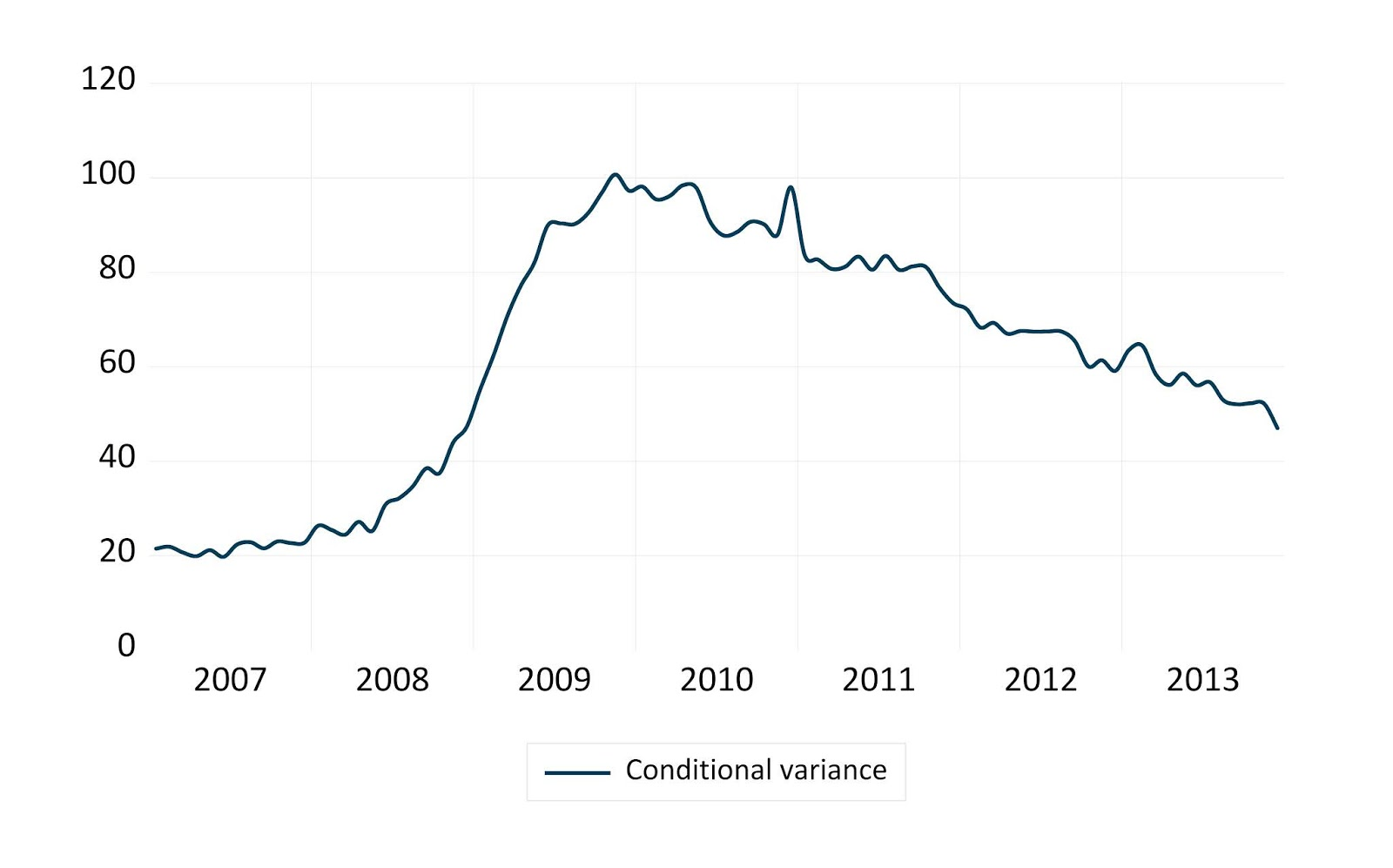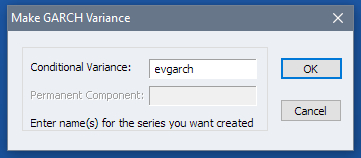Figure 4A: GARCH Conditional Variance of UNRATE Figure 4B: GARCH Conditional Variance Proc

### Data Analysis in Python

To estimate the GAS equivalent of this model we must first transfer our data over to Python. To do so, issue the following command in EViews:
    xopen(p)

This tells EViews to open an instance of Python within EViews and open up bi-directional communication. In fact you should see a new command window appear, titled Log: Python Output. Here you can issue commands into Python directly as if you had opened a Python instance at any command prompt. You can also send commands to Python using EViews command prompt. In fact, we will use the latter approach to import packages into our Python instance as follows:
    xrun "import numpy as np"
xrun "import pandas as pd"
xrun "import pyflux as pf"
xrun "import matplotlib.pyplot as plt"

For instance, the first command above tells eviews to issue the command import numpy as np in the open Python instance, thereby importing the NumPy package. In fact, all results will be echoed in the Python instance.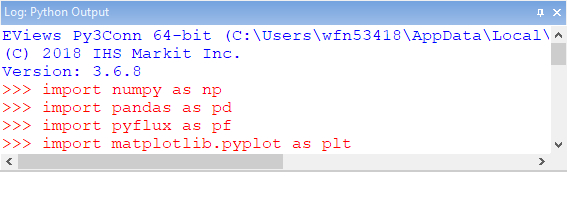Figure 5: Python Output Log

Next, transfer the UNRATE series over to Python by issuing the following command in EViews:
    xput(ptype=dataframe) unrate

The command above sends the series UNRATE to Python and transforms that data into a Pandas DataFrame object.

We now follow the PyFlux documentation and estimate the GAS model by issuing the following commands from EViews:
    xrun "model = pf.GAS(ar=1, sc=1, data=unrate, family=pf.Normal())"
xrun "fit = model.fit('MLE')"
xrun "fit.summary()"

The first command above tells PyFlux to create a GAS model object that has one autoregressive and one scaling parameter, sets $p(\cdot)$ to the Gaussian distribution, and uses the series UNRATE as $y_{t}$. In other words, the autoregressive and scaling parameters respectively corresponds to the coefficients $A$ and $B$ in the first section of this document. The second command tells Python to create a variable FIT which will hold the output from an estimated GAS model which uses maximum likelihood as the estimation technique. We display the output of this estimation by invoking the third command. In particular, we have the following estimates:
1. $\omega = 0.0027$
2. $A = 1.2973$
3. $B = 0.9994$
In fact, we can also obtain a distributional plot of the autoregressive coefficient $B$ across the period of estimation. To do this, invoke the following command within EViews:
    xrun "model.plot_z(, figsize=(15,5))"

The latter command tells Python to plot the distribution of the 2nd estimated coefficient (the AR coefficient) and to display a figure which is of size $15\times 5$ inches. This is the distribution of the evolution of $B$ and is not the time path of the estimated coefficient.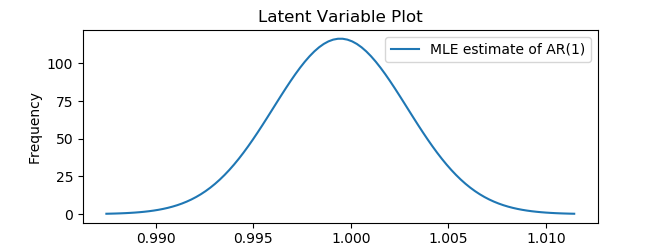Figure 6: Python GAS Distribution of AR Parameter

While we can obtain a distribution of the estimated parameters, unfortunately, PyFlux does not offer a way to extract the time path as a Python data object. Thankfully, we can recreate it manually and easily as a series in EViews.

### Back To EViews

To create the time path of the estimated GAS coefficient, we first need to transfer the coefficients from the estimated GAS model back into EViews. To do this, we invoke the following command in EViews:
    xget(name=gascoefs, type=vector) fit.results.x[0:3]

This tells Python to send the first three estimated coefficients back to EViews, and saves the result as a vector called GASCOEFS.

Next, create a new series in the workfile called GASGARCH by issuing the following command in the EViews:
    series gasgarch

Also, since this is an autoregressive process, we need to set an initial value for GASGARCH. We do this by setting the December 2006 observation to 0.7 -- the default value EViews uses to initialize its internal GARCH estimation. We do this by typing the following commands in EViews:
    smpl 2006M12 2006M12
gasgarch = 0.7

Next, we set the sample back to the period of interest and fill the values of GASGARCH using the GARCH formula with the coefficients from the GAS model. To do this, issue the following commands in EViews again:
    smpl 2007M01 @last
gasgarch = gascoefs(1) + gascoefs(3)*(unrate(-1)^2 - gasgarch(-1)) + gascoefs(2)*gasgarch(-1)

At last, we plot the GARCH conditional variance path from the internal estimation, EVGARCH along with the newly created series GASGARCH. We can do this programatically by issuing the following commands in EViews:
    plot evgarch gasgarch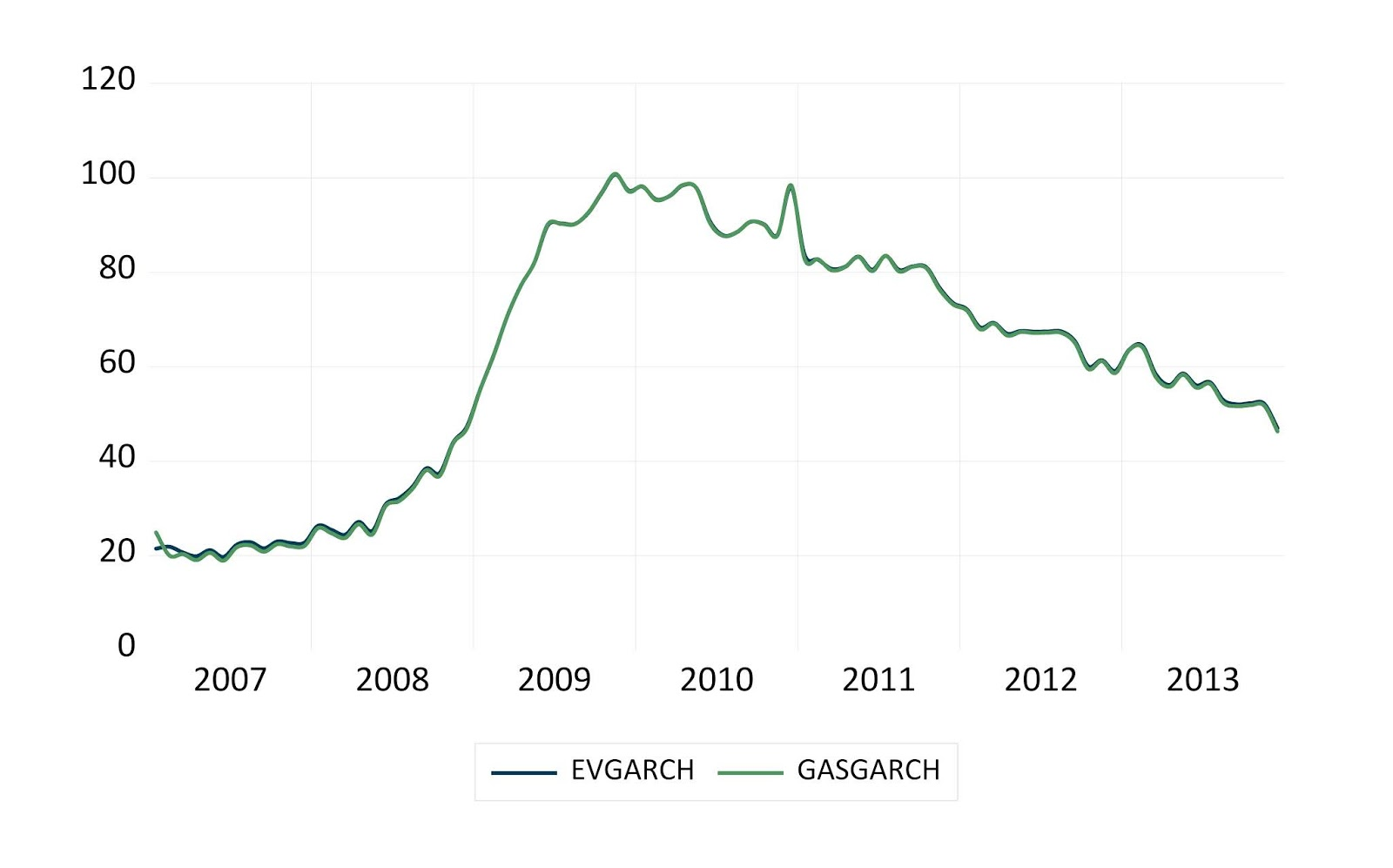Figure 7: GARCH Conditional Variance Comparison with GAS

It is clear that the two estimation techniques produce the same path despite having different estimates for the coefficients. At last, note that while GARCH models are estimated using maximum likelihood procedures, parameter estimates are typically numerically unstable and often fail to converge. This often requires a re-specification of the convergence criterion and / or a change in starting values. These drawbacks are also an issue with GAS models.